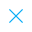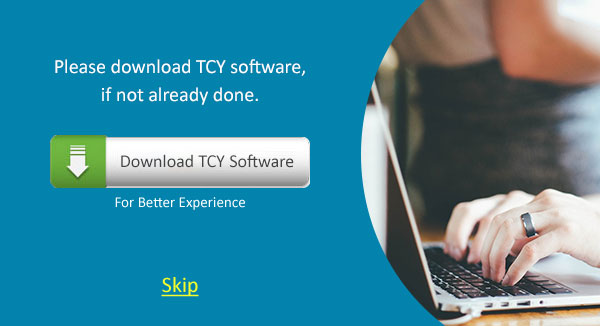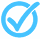Call Support +91-85588-96644

Keep me logged in
You can't leave Captcha Code empty
By submitting this form, you agree to the Terms & Privacy Policy.
ORTests given# FLT - 5 Mathematics (IX - 2018)

Topics Covered:Remainder and Factor TheoremProperties of Congruent TrianglesVolume of Figures Having Uniform Cross SectionApplication of Triangle InequalitiesCalculation of MeanConstruction of a Triangle when Angles; Sum and Difference of Sides are GivenDifferentiating and Identifying Rational and Irrational NumbersDistinguishing Rational and Irrational Numbers on the Basis of Decimal ExpansionEqual Chords of a Circle are Equidistant From the Center(s) and ConvFinding Solution of a Given Linear Equation

This pdf is currently unavailable.
Description:
This is the practice test of Mathematics (IX). It is helpful in the preparation of final exams.
Keywords: